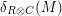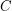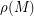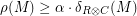### The formal definition of robustness, and of the problem

In all of the following definitions, the term "distance" refers to "relative Hamming distance".

Given a matrix, letdenote the distance fromto the nearest codeword of. Letdenote the average distance of a row ofto, and letdenote the average distance of a column ofto. Finally, letdenote the average ofand.

The tensor productis said to be-robust iff for every matrixwe have that.

The question is, under what conditions the tensor productis-robust for some constant.